• matlab获取图像像素坐标[已验证]
万次阅读 多人点赞
2018-05-23 09:29:01

1. impixelinfo

%例如:
%1.1 显示图片
mainf=imshow(data); %show the picture

%1.2 命令框中输入impixelinfo，点击图片即可

2. ginput

[x,y] = ginput(n)

%例如:

%显示图片

mainf=imshow(data); %show the picture

%↓返回的x值 ↓返回的x值                           ↓点选次数
[  x          ,      y           ]       =       ginput(1);

更多相关内容
• Matlab如何用鼠标获取图像像素值和坐标-test.m 如题 我是在一个GUI里，有两个axe，分别用来读取两个图片 我想要实现的是：图片在axe显示后，当鼠标在图片上移动，或者点击，可以得到图片的像素值和其在axe中的...
• Matlab如何用鼠标获取图像像素值和坐标-test.fig 如题 我是在一个GUI里，有两个axe，分别用来读取两个图片 我想要实现的是：图片在axe显示后，当鼠标在图片上移动，或者点击，可以得到图片的像素值和其在axe中...
• 获取图像内向量的所有像素坐标 我有一个强度/灰度图像，并且在该图像中选择了一个像素。我想从所有方向/角度从该像素开始发送矢量，并且我想对所有矢量求和触摸一个矢量的像素的所有强度的总和。在此步骤之后，我想...获取图像内向量的所有像素坐标

我有一个强度/灰度图像，并且在该图像中选择了一个像素。我想从所有方向/角度从该像素开始发送矢量，并且我想对所有矢量求和触摸一个矢量的像素的所有强度的总和。

在此步骤之后，我想绘制一个直方图，其中一个轴上的强度，另一个轴上的角度。我想我可以自己完成最后一步，但是我不知道如何在我的灰度图像中创建这些矢量以及如何获取矢量接触的像素的坐标。

我以前是用C ++做到的，这需要很多代码。我确信这可以在MATLAB中花费更少的精力来完成，但是我对MATLAB还是很陌生，因此将不胜感激，因为我在文档中没有发现任何帮助。

回答：

它可能不是解决问题的最佳方法，但是您可以使用一些代数来实现，这是如何...

我们知道以角度theta穿过点(a，b)的线的点斜率公式为：

y = tan(theta) * (xa) + b 因此，一个简单的想法是为所有const计算该线与y = const的交点，并读取该交点处的强度值。您将在所有角度重复此操作...

示例代码说明了这一概念：

%% input point = [128 128]; % pixel location I = imread('cameraman.tif'); % sample grayscale image %% calculations [rc] = size(I); angles = linspace(0, 2*pi, 4) + rand; angles(end) = []; clr = lines( length(angles) ); % get some colors figure(1), imshow(I), hold on figure(2), hold on for i=1:length(angles) % line equation f = @(x) tan(angles(i))*(x-point(1)) + point(2); % get intensities along line x = 1:c; y = round(f(x)); idx = ( yr ); % indices of outside intersections vals = diag(I(x(~idx), y(~idx))); figure(1), plot(x, y, 'Color', clr(i,:)) % plot line figure(2), plot(vals, 'Color', clr(i,:)) % plot profile end hold off

更多&回答...

展开全文• 本程序结合我的博客而仿真的，网址是：https://mp.csdn.net/postedit/82115829
• MATLAB图片点的像素坐标，可以精准的查看图片上每一个点的坐标，很精确，达到0.0001个像素！
• 但你可以用一些代数来做,继承人怎么样……我们知道通过角度θ的点(a,b)的线的Point-Slope formula是：y = tan(theta) * (x-a) + b因此,一个简单的想法是计算该线与所有常数的y = const的交点,并读取交点处的强度值....

它可能不是解决问题的最好方法,但你可以用一些代数来做,继承人怎么样……

我们知道通过角度θ的点(a,b)的线的

Point-Slope formula是：

y = tan(theta) * (x-a) + b

因此,一个简单的想法是计算该线与所有常数的y = const的交点,并读取交点处的强度值.你会为所有角度重复这个……

用于说明概念的示例代码：

%% input

point = [128 128]; % pixel location

I = imread('cameraman.tif'); % sample grayscale image

%% calculations

[r c] = size(I);

angles = linspace(0, 2*pi, 4) + rand;

angles(end) = [];

clr = lines( length(angles) ); % get some colors

figure(1), imshow(I), hold on

figure(2), hold on

for i=1:length(angles)

% line equation

f = @(x) tan(angles(i))*(x-point(1)) + point(2);

% get intensities along line

x = 1:c;

y = round(f(x));

idx = ( y<1 | y>r ); % indices of outside intersections

vals = diag(I(x(~idx), y(~idx)));

figure(1), plot(x, y, 'Color', clr(i,:)) % plot line

figure(2), plot(vals, 'Color', clr(i,:)) % plot profile

end

hold off

展开全文• 基于MATLAB图像处理技术的，二值化图像边缘提取，能方便的提取出二值化图像的边缘，并以坐标点的形式存储，后续应用。基于MATLAB图像处理技术的，二值化图像边缘提取，能方便的提取出二值化图像的边缘，并以坐标点的...
• 此功能针对图像处理应用程序，其中整数像素坐标很重要，而一个像素不能遗漏或重复。 在该视图中，使用圆角三角函数使用余弦调用生成的坐标是不够的。 中点圆算法就是答案。 重点是表现。 我们预先计算点的数量将由...
• 世界坐标、相机坐标、图像坐标、像素坐标的原理、关系，并用matlab仿真 照相机是日常生活中最常见的。它能把三维的空间图片等比例缩小投影在照片上，称为一个二维图像。 以下我们就讲一讲原理，并相应的进行matlab...

# 世界坐标、相机坐标、图像坐标、像素坐标的原理、关系，并用matlab仿真

照相机是日常生活中最常见的。它能把三维的空间图片等比例缩小投影在照片上，称为一个二维图像。

以下我们就讲一讲原理，并相应的进行matlab仿真。

在学之前，先要了解几个概念：

• 什么是世界坐标？
• 也就是真实世界的立体空间坐标，是一个三维坐标系
• Ow-XwYwZw ：世界坐标系,描述相机位置,单位m
• 什么是相机坐标？
• 根据透镜成像原理，将世界坐标在照相机内呈现，是一个三维坐标系
• Oc-XcYcZc  ：相机坐标系,光心为原点,单位m
• 什么是图像坐标？
• 将相机呈现的三维坐标投影到屏幕上，而建立的新坐标系，不含高程信息，是一个二维坐标系
• o-xy ：图像坐标系,原点为成像平面中点,单位mm
• 什么是像素坐标？
• 将投影的图像坐标离散抽样形成的做种图片，是一个二维的坐标系
• uv ：像素坐标系,原点为图像左上角,单位pixel

所以步骤流程就是：

• step1：将世界坐标转化为相机坐标，等比例缩小，外加旋转平移，称之为刚体变换；
• step2：将相机坐标转化为图像坐标，称为投影
• step3：将图像坐标离散抽样

模型如下图所示：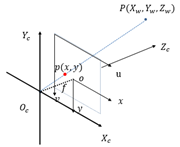## step1：构造rigbt()函数

世界坐标转化为相机坐标模型如下图所示：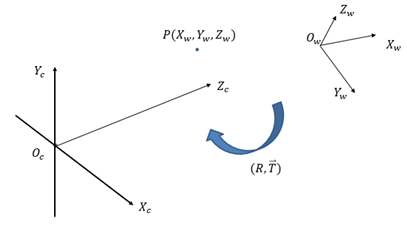从世界坐标系变换到相机坐标系属于刚体变换：即物体不会发生形变，只需要进行旋转和平移。

R：表示旋转矩阵

T：表示偏移向量

接下来分析旋转和平移

坐标轴绕z轴旋转 θ=Theta（希腊字母读法，编程用的到），其几何模型如下图所示：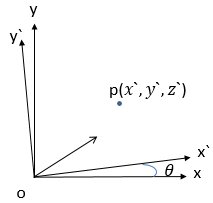公式满足：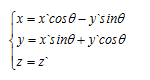矩阵形式为：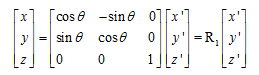同理，绕x、y轴可以写成：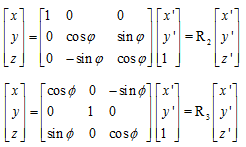所以刚体变化中旋转变换R=R1R2R3。

平移矩阵T，则刚体变换可以写成：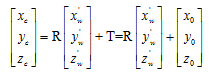进一步转化，可以写成4阶矩阵：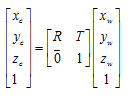R矩阵是一个3×3矩阵，T是一个3×1矩阵，RT是一个4×4矩阵。

matlab 仿真：

function [RT] = rigbt(Phi, Psi, Theta, x0, y0, z0)
%刚体变换函数:rigid body transformation
%输入参数：
%   φ=Phi       绕x轴转动的角度
%   ψ=Psi       绕y轴转动的角度
%   θ=Theta     绕z轴转动的角度
%输出参数：
%    RT 将坐标轴进行刚体变化（旋转+平移）成新的坐标轴
%
%% 程序
R1 = [1 0 0; 0 cos(Phi) sin(Phi); 0 -sin(Phi) cos(Phi)];%绕X轴旋转
R2 = [cos(Psi) 0 -sin(Psi); 0 1 0; sin(Psi) 0 cos(Psi)];%绕Y轴旋转
R3 = [cos(Theta) sin(Theta) 0;-sin(Theta) cos(Theta) 0; 0 0 1 ];%绕Z轴旋转
R = R3 * R1 * R2;%旋转矩阵（刚体变换一部分）
T = [x0; y0; z0];%平移矩阵
RT=[R T;0 0 0 1];%刚体变换矩阵
return


## step2：构造proj()函数

相机坐标投影成图像坐标，几何模型如下图所示：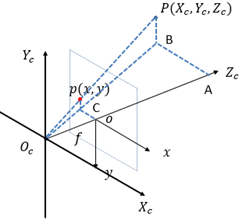几何关系（相似）满足：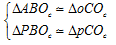即：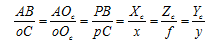代入：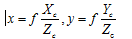写成矩阵形式：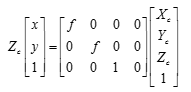matlab 仿真：

function [Projection_Matrix] = proj(f)
%相机坐标投影(projection)成图像坐标
%输入参数
%   f                   相机焦距（数，cm）
%输出参数
%   Projection_Matrix   投影成像（矩阵）
Projection_Matrix = [f 0 0 0; 0 f 0 0; 0 0 1 0];
return

## step3：构造pixel()函数

xoy是图像坐标； uo_{uv}v是像素坐标； (u0,v0)是像素坐标的中心； p(x,y)是图像中的任意一点。

模型如下：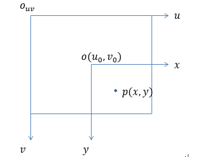将x、y分成n份，每份长度为dx、dy。

则几何公式为：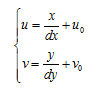转化为矩阵形式：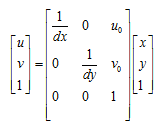matlab仿真:

function [Pixel_Matrix] = pixel(dx,dy,u0,v0)
%图像坐标离散化，转化为像素坐标
%输入参数：
%   dx      x轴方向上分辨率，像素大小
%   dy      y轴方向上分辨率，像素大小
%   (u0,v0) 参考坐标，图像平面中心
if nargin==2
u0 = 0;
v0 = 0;
end
Pixel_Matrix = [1/dx 0 u0; 0 1/dy v0; 0 0 1];
return


## step4：前三步整合

公式中， fx=f/dx,fy=f/dy。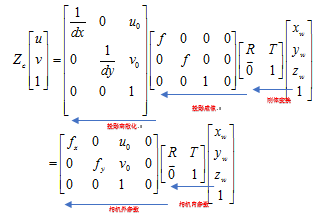## matlab 主程序

% 将世界坐标转化为像素坐标的参数
%% 程序
clear all;close all; clc;
% 参数
Phi = pi/4;%绕x轴旋转角度
Psi = pi/4;%绕y轴旋转角度
Theta = pi/4;%绕z轴旋转角度
x0 = 0;%x平移量
y0 = 0;%y平移量
z0 = 0;%z平移量
f = 35e-3;%35mm相机
dx = 0.026;%一个像素的长
dy = 0.026;%一个像素的宽
u0 = 0;%图像平面中心
v0 =0;%图像平面中心

%% step1:将世界坐标转化为相机坐标，等比例缩小，外加旋转平移(刚体变换)
RT = rigbt(Phi, Psi, Theta, x0, y0, z0);%刚体变换矩阵

%% step2:将相机坐标转化为图像坐标(投影)
Projection_Matrix = proj(f);%投影矩阵

%% step3:将图像坐标离散抽样
Pixel_Matrix = pixel(dx,dy,u0,v0);%1像素＝0.635厘米÷24≈0.026458厘米
%% 数据整合
Camera_Internal_Parameters = Pixel_Matrix * Projection_Matrix;%相机内参数
Camera_External_Parameters = RT;%相机外参数
%清除不用的变量
clearvars -except Camera_Internal_Parameters Camera_External_Parameters
%相机参数
Camera_Parameters = Camera_Internal_Parameters*Camera_External_Parameters
clearvars -except Camera_Parameters%清除变量


仿真结果：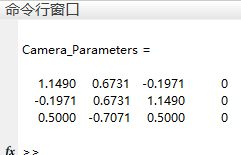## 扩展：

实际应用中，往往将上面的主程序main制作成一个相机参数函数camera_ parameters()，更有利于调用。可以用下面相机参数函数一个代替上面的main程序。

matlab 仿真

function [Camera_Parameters] = camera_parameters()
% 将世界坐标转化为像素坐标的参数(相机参数矩阵)
% 参数,可以省，省去的话，必须设置相应的入参
Phi = pi/4;%绕x轴旋转角度
Psi = pi/4;%绕y轴旋转角度
Theta = pi/4;%绕z轴旋转角度
x0 = 0;%x平移量
y0 = 0;%y平移量
z0 = 0;%z平移量
f = 35e-3;%35mm相机
dx = 0.026;%一个像素的长
dy = 0.026;%一个像素的宽
u0 = 0;
v0 =0;

%% step1:将世界坐标转化为相机坐标，等比例缩小，外加旋转平移(刚体变换)
RT = rigbt(Phi, Psi, Theta, x0, y0, z0);%刚体变换矩阵

%% step2:将相机坐标转化为图像坐标(投影)
Projection_Matrix = proj(f);%投影矩阵

%% step3:将图像坐标离散抽样
Pixel_Matrix = pixel(dx,dy,u0,v0);%1像素＝0.635厘米÷24≈0.026458厘米
%% 数据整合
Camera_Internal_Parameters = Pixel_Matrix * Projection_Matrix;%相机内参数
Camera_External_Parameters = RT;%相机外参数
%相机参数
Camera_Parameters = Camera_Internal_Parameters*Camera_External_Parameters;


仿真结果：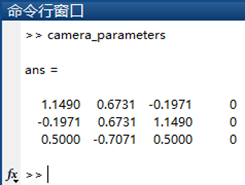注：

给学matlab的人，包括我自己一个勉励:路漫漫其修，吾上下而求索

展开全文世界坐标系
• ## Matlab查看像素坐标

千次阅读 2020-10-27 10:37:24
matlab弹出的figure中随鼠标移动实时显示该处坐标像素值，在command window中输入IMPIXELINFO即可，在当前图像中查看信息
• 此代码将像素位置转换为其质心坐标。 它适用于 DICOM 多切片图像，其中每个体素都是一个立方体。 该函数取 INPUT：VoxDim=体素尺寸，单位为微米； name_mask='MaskName.dcm'。 它提供每个像素质心的 xyz 坐标作为 ...
• lz 这个应当有多种思路因为只要求最大8个值及对应坐标 所以用排序 过了点设图像前8个值所组成的1-D数组为MAX_value，对应1-D坐标数组为MAX_coor_x，MAX_coor_y,threshold为MAX_value的中各元素的最小值，A为对应图像...
• 在不同的仿真工具和不同格式的图像中，表示方式有些区别，但常用的一般以下三种：矩阵坐标系、直角坐标系、像素坐标系。 1、矩阵坐标系 按照行列顺序定位数据。矩阵坐标系原点定位在左上角，图像A(i,j)，i表示行，...矩阵
• 它显示了如何从轴坐标转换为图像像素坐标.如果使用除默认1：width和1：height之外的自定义XData / YData位置绘制图像,此功能尤其有用.在下面的示例中,我在x / y方向上移动了100和200像素.function imageExample()%# ...
• ## matlab鼠标定位图像中像素点的坐标

万次阅读 多人点赞 2020-09-05 13:58:03
命令窗口输入： mainf=imshow('C:/XXXXXXXX/Desktop/1.png'); impixelinfo; 在图片上移动鼠标即可查看。 如图：
• 利用Matlab读取图片各个像素的RGB颜色值，然后在Excel中将各像素RGB颜色对应地填充到单元格中。
• Java基础-接口看下图实现如下接口和类，并完成Adventure中的主方法package hanqi; public interface CanSwim { void swim(); } package hanqi; public interface CanFly { pu ...台湾辅仁大学的python教程笔记散记,...
• 根据坐标获取像素值 P=impixel(im,r,c) r:行坐标 c:纵坐标 P：返回的RGB值 im=imread('C:\Users\14372\Desktop\Music_pictures\图片\梦.png'); r=[40 60 80]; c=[100 120 140]; figure; imshow(im); p=impixel(im,r,...
• 可通过改变代码中图片的输入路径，输入任意图片，并读取出图片上任意点的亚像素坐标。 可通过改变代码中图片的输入路径，输入任意图片，并读取出图片上任意点的亚像素坐标
• 输入 clear all； RGB = imread（‘图片名.格式’）； r = [x1 y1 z1];%指定像素点的横坐标 ...p = impixel(RGB,r,c）%用impixel（）函数来返回RGB图像的横纵坐标对应的像素点的值 输出： p = a b c d e f g h i ...
• 原创Matlab提取圆点中心坐标-circle.rar 首先感谢论坛的资料，让我少走了弯路。 circle.rar 名称：提取圆点中心坐标 测试图像：背景为黑色，圆点为白色。测试图像有五个圆点。 功能：提取...
• 旋转图像后，根据像素坐标生成 2D 多项式，因此环形图像的赤道是水平的并定义了原点。
• 很好的提取指定图像中指定颜色的像素坐标的c++代码...

# matlab获取图像像素坐标matlab 订阅